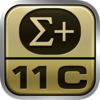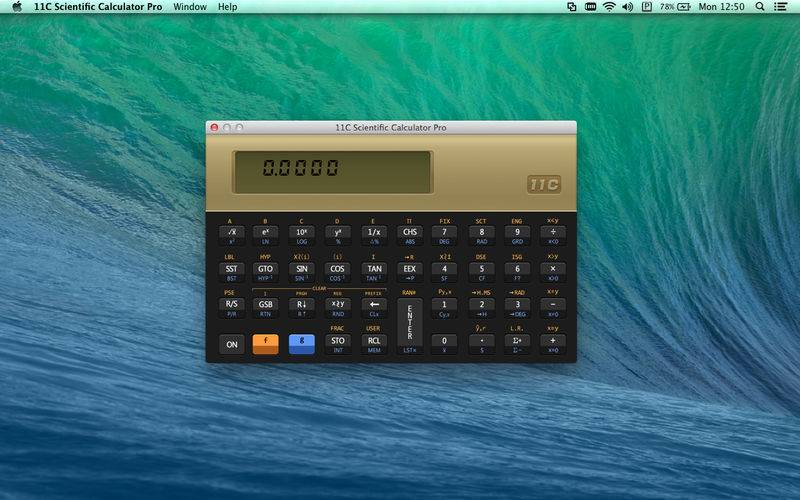# 11C Scientific Calculator Pro

OS X 10.6.6

50

25

11C Scientific Calculator Pro is the most accurate and precise emulator of the HP 11C RPN scientific programmable calculator. It uses the same RPN (Reverse Polish Notation) entry system and supports the same mathematical functions as the HP 11C Scientific Calculator. **** Features **** • Hyperbolic and inverse hyperbolic trig functions. • Probability (combinations and permutations). • Factorial, absolute, integer & fractional. • Random number generator. • Index register for indirect operations. • User mode to run program labels. • Cumulative statistical analysis & Linear regression. • Hour minute second conversion. • Polar, rectangular conversion. • RPN entry. • Programmable.